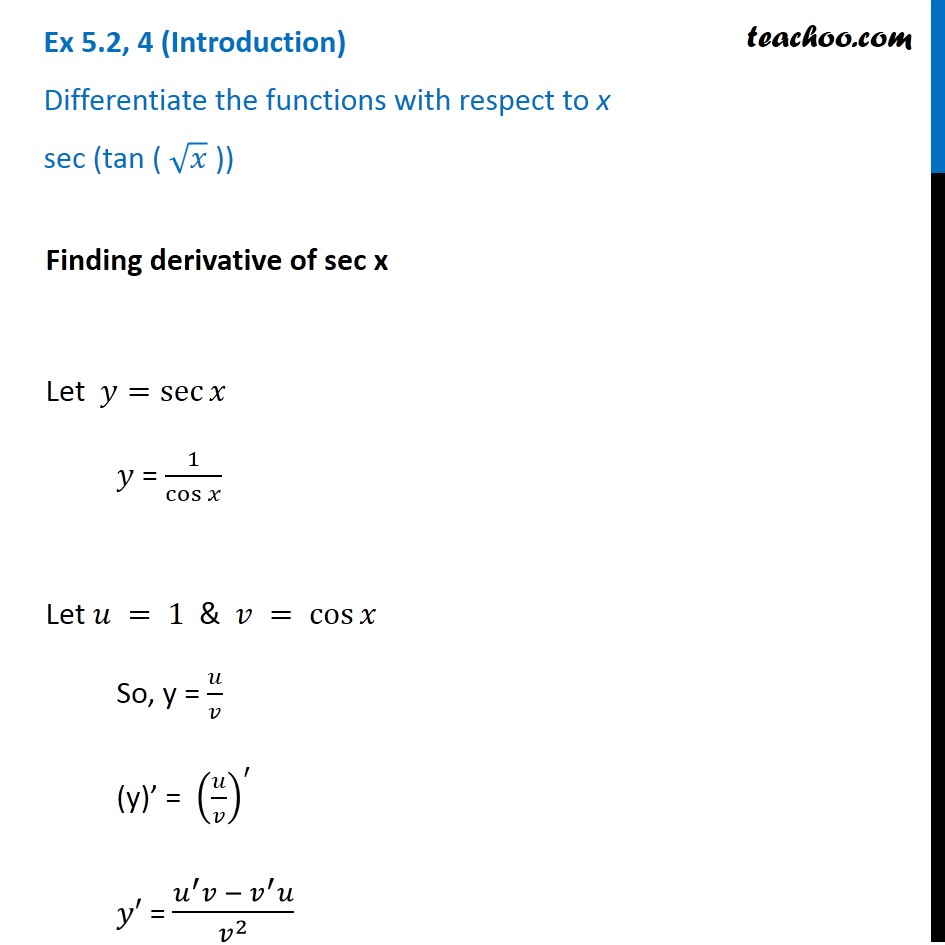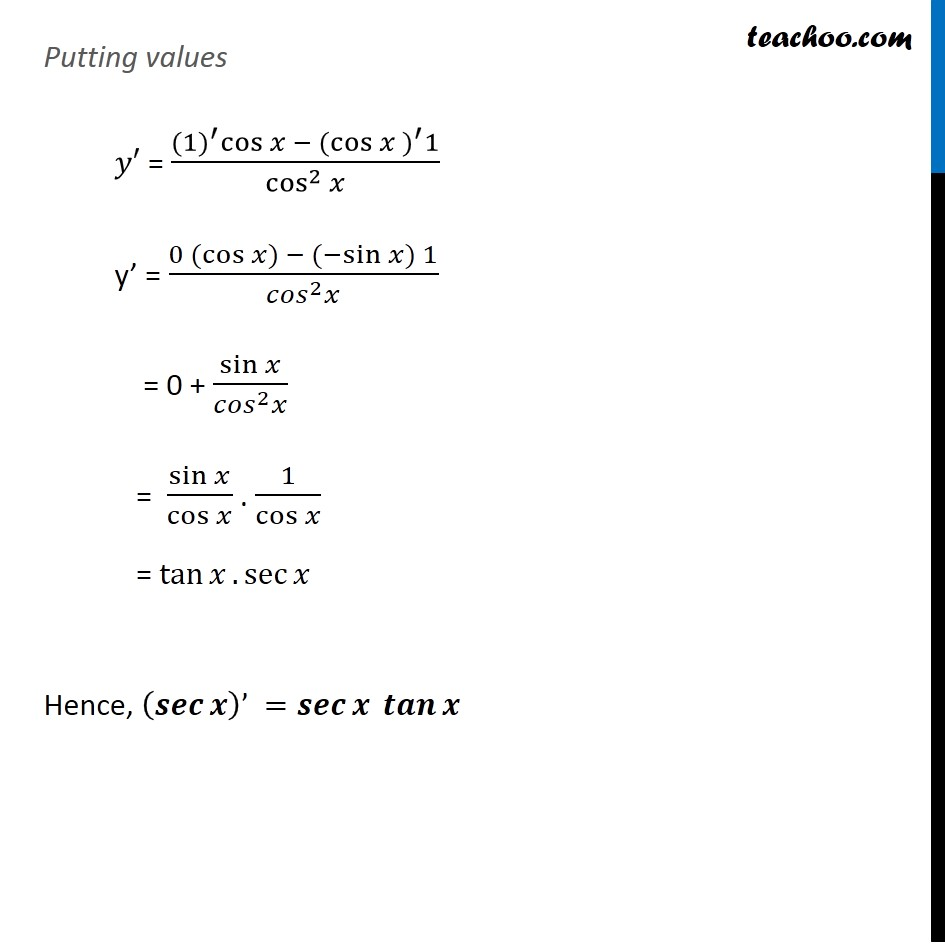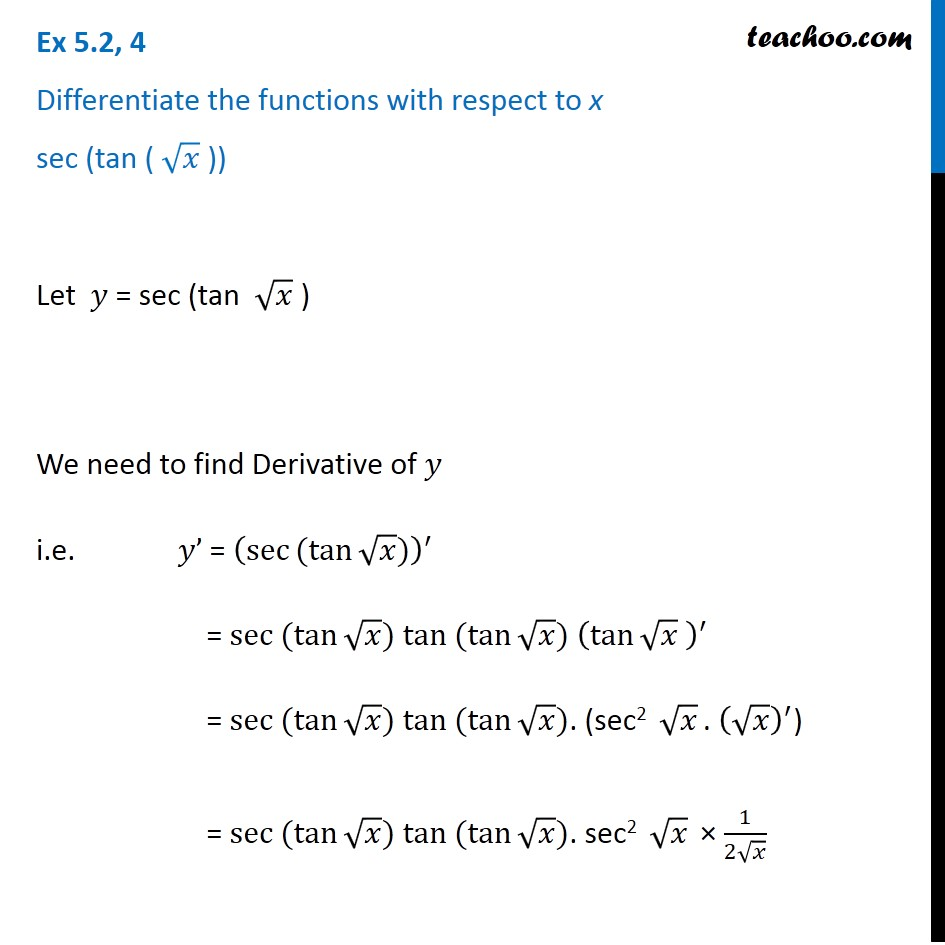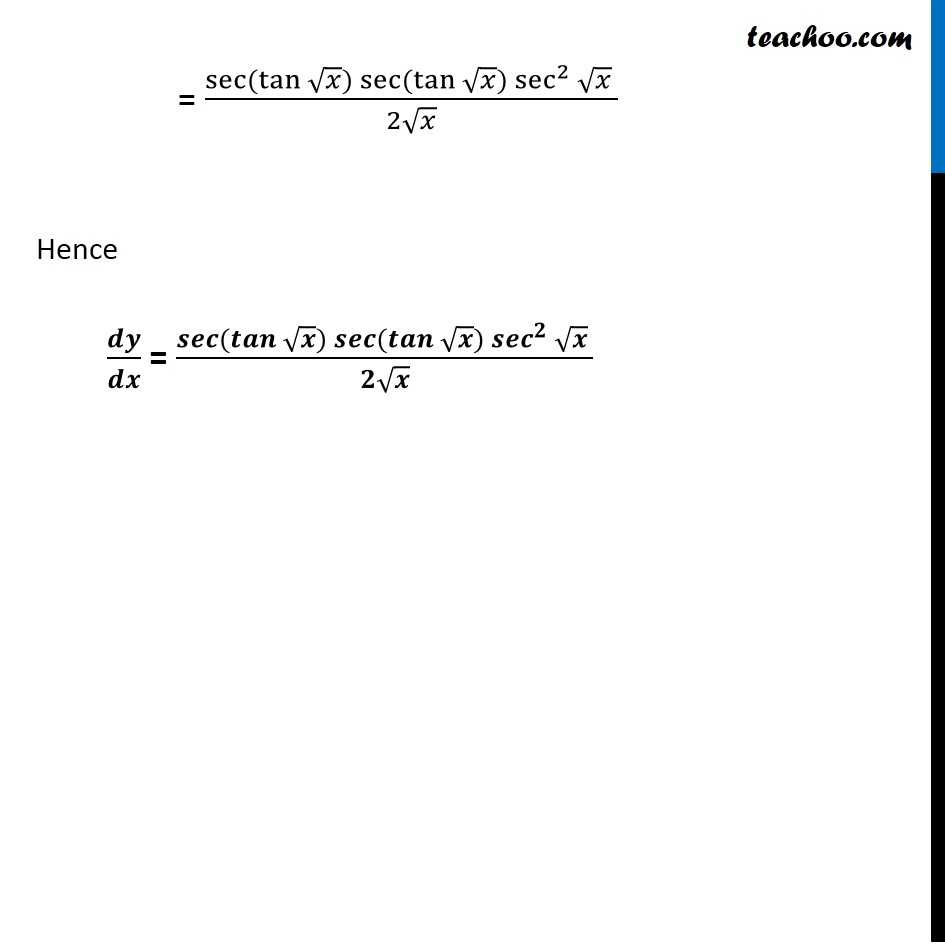Check Full Chapter Explained - Continuity and Differentiability - Continuity and Differentiability Class 121. Chapter 5 Class 12 Continuity and Differentiability
2. Serial order wise
3. Ex 5.2

Transcript

Ex 5.2, 4 (Introduction) Differentiate the functions with respect to x sec (tan ( √𝑥 )) Finding derivative of sec x Let 𝑦=sec⁡𝑥 𝑦 = 1/cos⁡𝑥 Let 𝑢 = 1 & 𝑣 = cos⁡𝑥 So, y = 𝑢/𝑣 (y)’ = (𝑢/𝑣)^′ 𝑦′ = (𝑢^′ 𝑣 − 𝑣^′ 𝑢)/𝑣^2 Putting values 𝑦′ = (〖(1)〗^′ cos⁡𝑥 − 〖(cos⁡〖𝑥 〗)〗^′ 1)/cos^2⁡𝑥 y’ = (0 〖(cos〗⁡〖𝑥)〗 − (〖−sin〗⁡〖𝑥) 1〗)/(〖𝑐𝑜𝑠〗^2 𝑥) = 0 + sin⁡𝑥/(〖𝑐𝑜𝑠〗^2 𝑥) = sin⁡𝑥/cos⁡𝑥 . 1/cos⁡𝑥 = tan⁡𝑥.sec⁡𝑥 Hence, (𝒔𝒆𝒄⁡𝒙 )’ =𝒔𝒆𝒄⁡𝒙 𝒕𝒂𝒏⁡𝒙 Ex 5.2, 4 Differentiate the functions with respect to x sec (tan ( √𝑥 )) Let 𝑦 = sec (tan √𝑥 ) We need to find Derivative of 𝑦 i.e. 𝑦’ = (sec⁡〖〖(tan〗⁡√𝑥)〗 )^′ = 〖sec 〗⁡〖(tan⁡√𝑥)〗 〖tan 〗⁡〖(tan⁡√𝑥)〗 (tan⁡√𝑥 )^′ = 〖sec 〗⁡〖(tan⁡√𝑥)〗 〖tan 〗⁡〖(tan⁡√𝑥)〗. ("sec2 " √𝑥 " . " (√𝑥)^′) = 〖sec 〗⁡〖(tan⁡√𝑥)〗 〖tan 〗⁡〖(tan⁡√𝑥)〗. sec2 " " √𝑥 × 1/(2√𝑥) = (sec⁡〖(tan⁡√𝑥 〗)sec⁡〖(tan⁡√𝑥 〗)sec^2⁡√𝑥 )/(2√𝑥) Hence 𝒅𝒚/𝒅𝒙 = (𝒔𝒆𝒄⁡〖(𝒕𝒂𝒏⁡√𝒙 〗)𝒔𝒆𝒄⁡〖(𝒕𝒂𝒏⁡√𝒙 〗)〖𝒔𝒆𝒄〗^𝟐⁡√𝒙 )/(𝟐√𝒙)

Ex 5.2